Electron. J. Differential Equations, Vol. 2018 (2018), No. 168, pp. 1-54.

### Analytic solutions and complete markets for the Heston model with stochastic volatility Benedicte Alziary, Peter Takac

Abstract:
We study the Heston model for pricing European options on stocks with stochastic volatility. This is a Black-Scholes-type equation whose spatial domain for the logarithmic stock price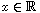and the variance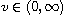is the half-plane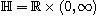. The volatility is then given by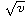. The diffusion equation for the price of the European call option p = p(x,v,t) at time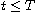is parabolic and degenerates at the boundary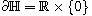as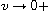. The goal is to hedge with this option against volatility fluctuations, i.e., the function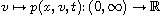and its (local) inverse are of particular interest. We prove that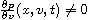holds almost everywhere in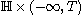by establishing the analyticity of p in both, space (x,v) and time t variables. To this end, we are able to show that the Black-Scholes-type operator, which appears in the diffusion equation, generates a holomorphic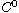-semigroup in a suitable weighted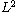-space over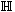. We show that the-semigroup solution can be extended to a holomorphic function in a complex domain in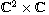, by establishing some new a~priori weighted-estimates over certain complex "shifts" offor the unique holomorphic extension. These estimates depend only on the weighted-norm of the terminal data over(at t=T).

Submitted August 22, 2018. Published October 11, 2018.
Math Subject Classifications: 35B65, 91G80, 35K65, 35K15.
Key Words: Heston model; stochastic volatility; Black-Scholes equation; European call option; degenerate parabolic equation; terminal value problem; holomorphic extension; analytic solution.

Show me the PDF file (641 KB), TEX file for this article.Bénédicte Alziary Toulouse School of Economics, I.M.T. Université de Toulouse - Capitole 21 Allées de Brienne F-31000 Toulouse Cedex, France email: benedicte.alziary@ut-capitole.fr Peter Takác Universität Rostock Institut für Mathematik Ulmenstrase~69, Haus 3 D-18057 Rostock, Germany email: peter.takac@uni-rostock.de